# MCQs on Electric Circuits

##### Page 58 of 63. Go to page 1 2 3 4 5 6 7 8 9 10 11 12 13 14 15 16 17 18 19 20 21 22 23 24 25 26 27 28 29 30 31 32 33 34 35 36 37 38 39 40 41 42 43 44 45 46 47 48 49 50 51 52 53 54 55 56 57 58 59 60 61 62 63
01․ In a 3 - φ delta connection, two-wattmeter method is used to measure the power; if the two watt meters read 28.13 kW and 13.31 kW, the power factor of connection will be
0.85.
0.9.
0.6.
0.4.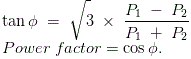02․ In above question, if circuit efficiency is 90 %. What will be output power ?
30 kW.
37.3 kW.
35.6 kW.
40 kW.

Output power = efficiency X ( P1 + P2 ).

03․ Consider the following circuit :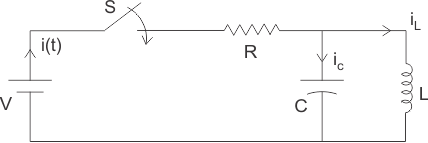Switch is closed at t = 0 Find i(0 +)
0.
(V*L) / (R*C)
V/R.
V / {R + (sL + 1 / Cs)}.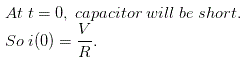04․ In question no.3, which of the following is true ?
ic (0+) = i(0+).
i(0+)= iL(0+).
iL(0) = ic (0).
None of these.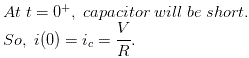05․ A 10 Ohm resistor has power rating of 1W. Find the maximum current rating.
0.1 A.
0.01 A.
0.316 A.
1 A.

I2R = 1; I = √0.1 = 0.316 A

06․ What would be the power given by source V2?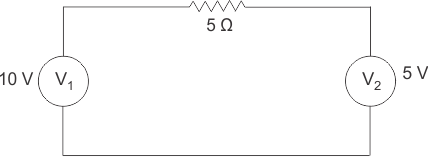- 5 W.
5 W.
10 W.
0 W.

Among two voltage source, only 10 V source will deliver power to 5 V voltage source.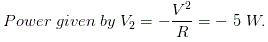07․ Consider the following circuit.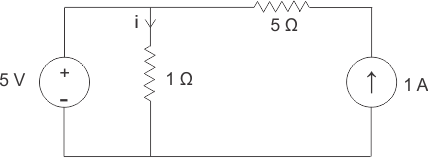What is the value of i ?
- 5 A.
5 A.
6 A.
12 A.

Voltage source will offer zero resistance path to current. So net current in 1 Ω resistance depends on only voltage source. So current = 5 X i = 5 A.

08․ In above question, power given in the 5 V source is
20 W.
25 W.
30 W.
5 W.

Source current = 5 - 1 = 4 Amp. Power given by the 5 V source = 5 X 4 = 20 W.

09․ Total power consumed in the circuit below is 10 W. Find X.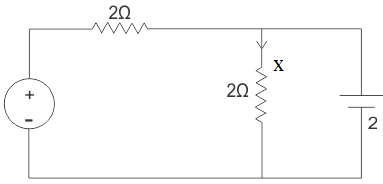1 A.
2 A.
3 A.
None of these.

Total power consumed = x2 X 2 + 22/2 ∴ 10 W = 2x2 + 2 Therefore, x = 2 A.

10․ If Vout = 1 V for 1 kHz input then what would be Vout for 10 kHz for same input ?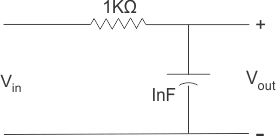0.1 V .
0.01 V.
1 V.
None of these.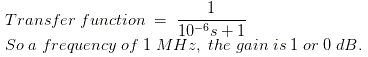<<<5657585960>>>